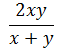Average Questions for bank and all sarkari naukri examinations.

## Average Questions for IBPS and SSC Exams

Basic Concepts of Average:

What is an average?

In simple terms, averages usually refer to the sum of given numbers divided by the total number of terms listed.

Averages = (sum of all terms)/ number of terms

The main term of average is equal distribution of a value among all which may distribute persons or things. We obtain the average of a number using formula that is sum of observations divided by Number of observations.

Important Formula for Average:

Find the Average Speed

• If a person travels a distance at a speed of x km/hr and the same distance at a speed of y km/hr then the average speed during the whole journey is given by-• If a person covers A km at x km/hr and B km at y km/hr and C km at z km/hr, then the average speed in covering the whole distance is-• The average of the series which is in A.P. can be calculated by ½(first + last term)
• If the average of n numbers is A and if we add x to each term then the new average will be = (A+ x).
• If the average of n numbers is A and if we multiply p with each term then the new average will be = (A x p).
• When a person leaves the group and another person joins the group in place of that person then-

Case1: If the average age is increased,
Age of new person = Age of separated person + (Increase in average × total number of     persons)

Case2: If the average age is decreased,
Age of new person
= Age of separated person – (Decrease in average × total number of      persons)

• When a person joins the group-
Case1: In case of increase in average                                                                        Age of new member = Previous average + (Increase in average × Number of members including new member)Case2: In case of decrease in average                                                                       Age of new member = Previous average – (Decrease in average × Number of members including new member)
• In the Arithmetic Progression there are two cases when the number of terms is odd and second one is when number of terms is even. So when the number of terms is odd the average will be the middle term. when the number of terms is even then the average will be the average of two middle terms.

From the Average topic, you will surely score better using these important formulas, and this average section mainly seen in all the bank exams such as SBI PO, SBI Clerck, IBPS PO, and specialist officers and also in all sarkari naukri examinations such as SSC CGL. SSC CHSL , RRB NTPC etc. So, here are some questions and quiz on Average, using the above rules, now test your knowledge on Average topic.

### Average Questions and Quiz:

 BOOK COVER NAME OF BOOK ONLINE PURCHASEFast Track Objective Arithmetic by Rajesh VermaQuantitative Aptitude for Competitive Examinations by R.S. AggarwalAdvance maths for General Competitions by Rakesh YadavFriends this is it for Quantitative Aptitude Preparation. If you have any queries/questions regarding Best Books for any Section, Feel free to ask them in the comments section below. And we will be glad to answer them for you.

QUANTITATIVE APTITUDE FREE QUIZES

#### 1 COMMENT

1. question no. 1 : A Batsman makes a score of 98 runs in the 20th inning and thus increases his average by 3.
means if he was having an average of x runs till his 19th inning then it becomes x + 3 after 20th inning… that implies (19x + 98)/20 = (x + 3) => x = 38 please explain i’m confused